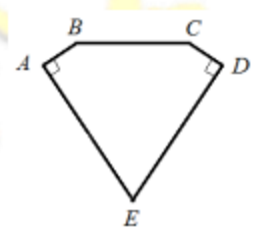###### back to index | new

If the middle term of three consecutive integers is a perfect square, then the product of these three numbers is called a $\textit{beautiful}$ number. What is the greatest common divisor of all the $\textit{beautiful}$ numbers?

Find the largest 7-digit integer such that all its 3-digit subpart is either a multiple of 11 or multiple of 13.

Find a $4$-digit square number $x$ such that if every digit of $x$ is increased by 1, the new number is still a perfect square.

If $a+b=\sqrt{5}$, compute $\frac{a^2 -a^2b^2 + b^2 +2ab}{a+ab+b}$.

What is the units digit of $-1\times 2008 + 2 \times 2007 - 3\times 2006 + 4\times 2005 +\cdots-1003\times 1006 + 1004 \times 1005$?

If $-1 < a < b < 0$, then which relationship below holds? $(A)\quad a < a^3 < ab^2 < ab \qquad (B)\quad a < ab^2 < ab < a^3 \qquad (C)\quad a< ab < ab^2 < a^3 \qquad (D) a^3 < ab^2 < a < ab$

Let point $A$ and $B$ represent real numbers $a$ and $b$, respectively. If $A$ and $B$ lay on different sides of the origin $O$, and $|a - b| = 2016$, $AO = 2BO$, what is the value of $a+b$?

If $a+b=\sqrt{5}$, compute the value of $\frac{a^2 - a^2b^2 + b^2 +2ab}{a+ab+b}+ab$.

If $AE=DE=5$, $AB=CD$, $BC=4$, $\angle{E}=60^\circ$, $\angle{A}=\angle{D}=90^\circ$, then what is the area of the pentagon $ABCDE$?How many solutions does the following system have? $$\left\{ \begin{array}{ll} \lfloor x \rfloor + 2y &= 1\\ \lfloor y \rfloor + x &=2 \end{array} \right.$$ Where $\lfloor x \rfloor$ and $\lfloor y \rfloor$ denote the largest integers not exceeding $x$ and $y$, respectively.